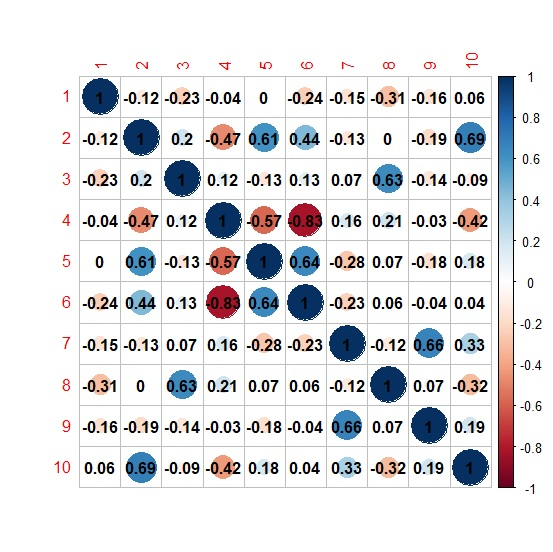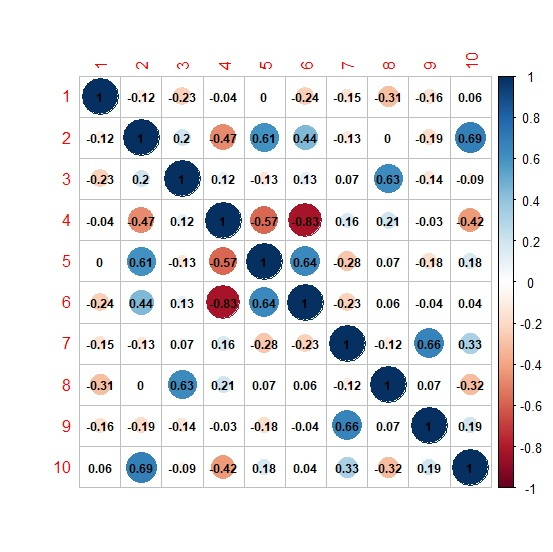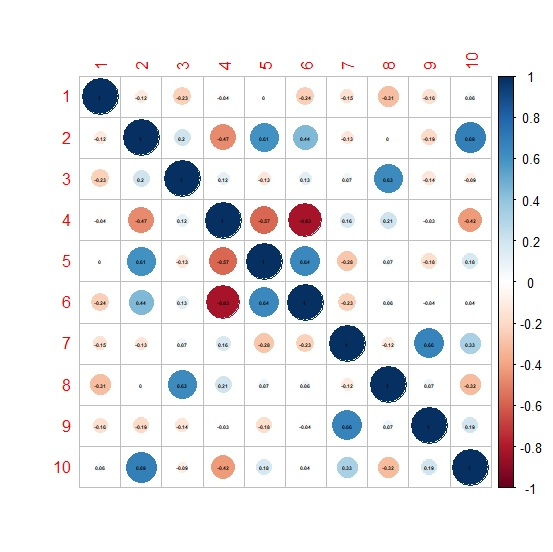# How to change the size of correlation coefficient value in correlation matrix plot using corrplot in R?

The correlation coefficient value size in correlation matrix plot created by using corrplot function ranges from 0 to 1, 0 referring to the smallest and 1 referring to the largest, by default it is 1. To change this size, we need to use number.cex argument. For example, if we want to decrease the size to half then we can use number.cex = 0.5.

## Example

Consider the below matrix −

Live Demo

set.seed(99)
M<-matrix(rpois(100,10),ncol=10)
M

## Output

      [,1] [,2] [,3] [,4] [,5] [,6]  [,7]  [,8]  [,9] [,10]
[1,]    10  10   14   14    7    9    14    14    13    10
[2,]    11   8    9    13   9    9     5    12     7     6
[3,]    10  12    6    11   5    8    10     4    11    15
[4,]    11   7   12     9   5   10     7    10    10     9
[5,]     8   8    8    16   8    6     7    14    12     8
[6,]    10   4    5    10   6    8    14     9    14    11
[7,]     7  12    9     4   14  18     7    12    12     9
[8,]    11  16    8     6   18  12     9     9    10    16
[9,]     5   9   11    13   8   10    12    10    10     9
[10,]    7  14   13     9   7   10    10    15    11    16

library(corrplot) > corrplot(cor(M),addCoef.col="black")

## OutputChanging the size of correlation coefficient value to 0.75 −

corrplot(cor(M),addCoef.col="black",number.cex=0.75)

## OutputChanging the size of correlation coefficient value to 0.30 −

> corrplot(cor(M),addCoef.col="black",number.cex=0.30)

## Output